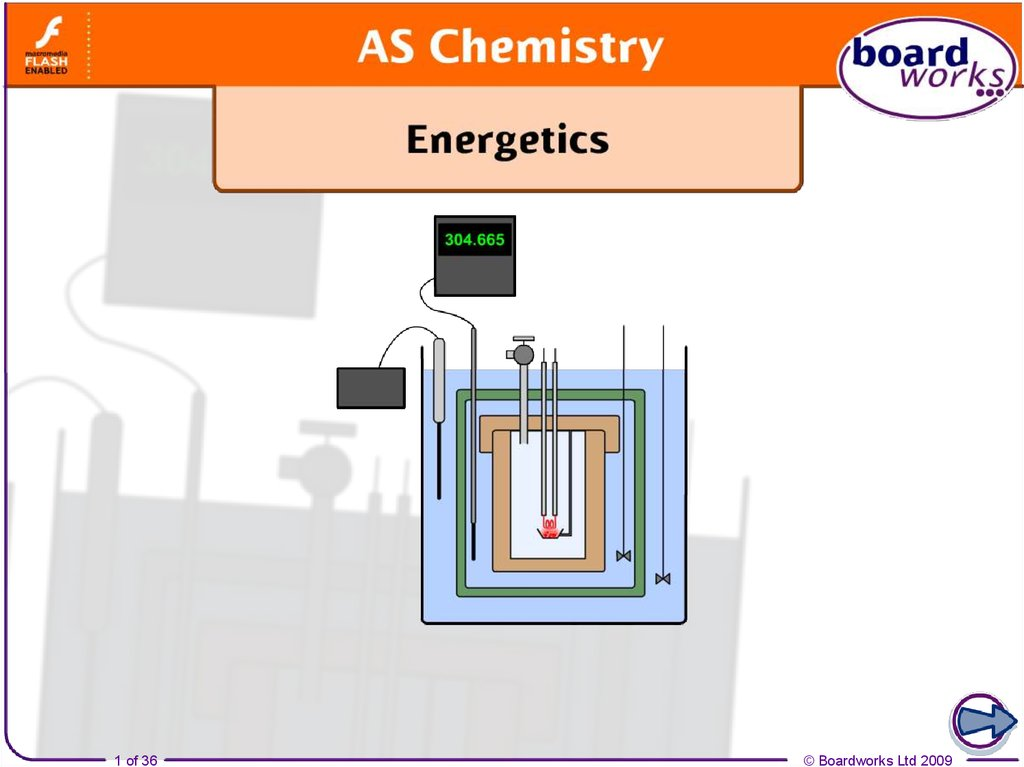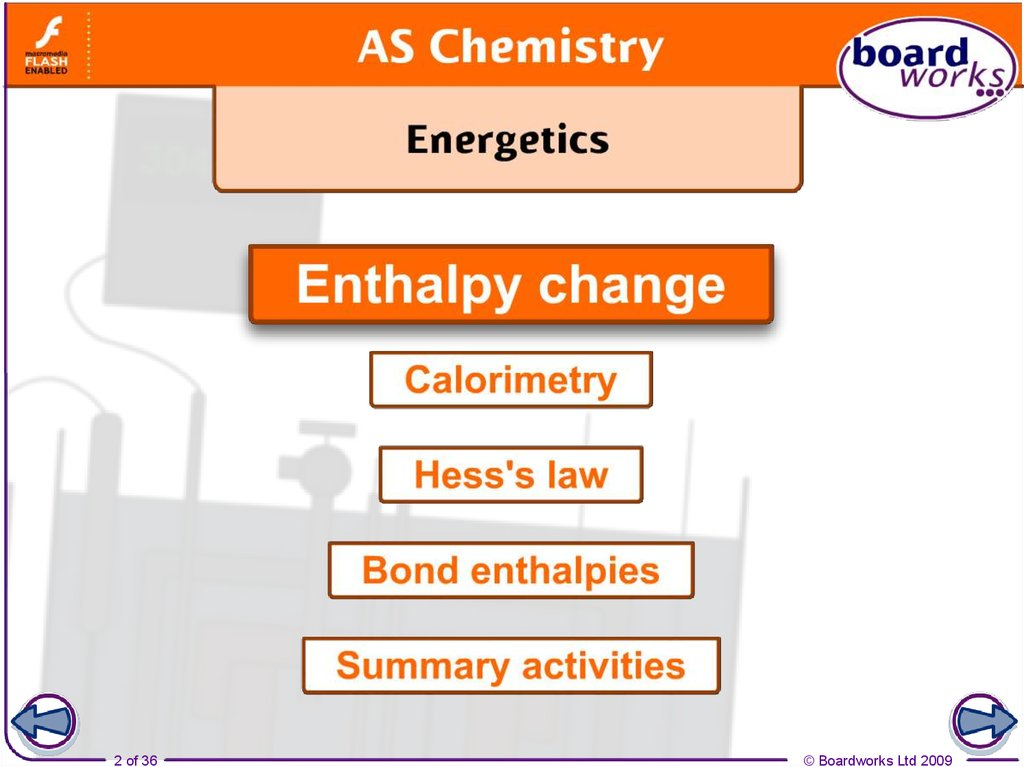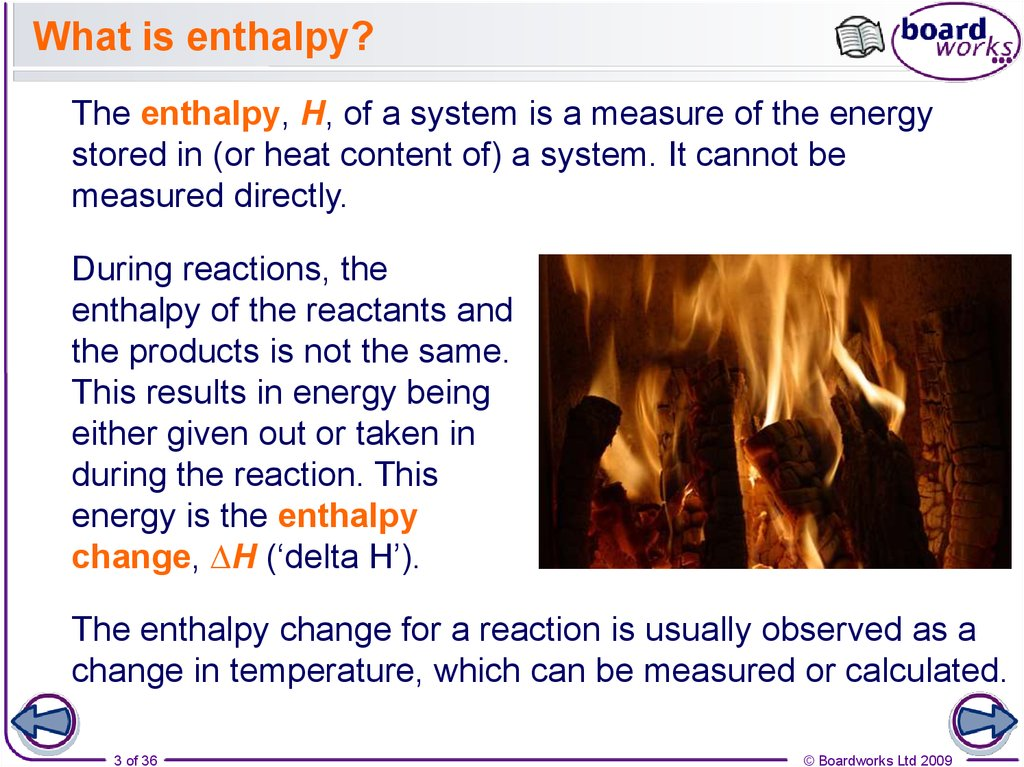# What is enthalpy?

1 of 36

2 of 36

## 3. What is enthalpy?

The enthalpy, H, of a system is a measure of the energy
stored in (or heat content of) a system. It cannot be
measured directly.
During reactions, the
enthalpy of the reactants and
the products is not the same.
This results in energy being
either given out or taken in
during the reaction. This
energy is the enthalpy
change, ∆H (‘delta H’).
The enthalpy change for a reaction is usually observed as a
change in temperature, which can be measured or calculated.
3 of 36

## 4. Enthalpy changes

The enthalpy change of a reaction is the heat energy
exchange with its surroundings at constant pressure.
Enthalpy is the energy content of the reactants and is
given the symbol H.
In science, change is represented by the upper case
Greek letter delta, ∆.
Therefore, enthalpy change is represented by ∆H. It has
the units kilojoules per mole (kJ mol-1).
Standard enthalpy changes are measured at a standard
pressure of 100 kPa and temperature of 298 K. Standard
enthalpy changes are represented by ∆Hө298 but this is
usually shortened to ∆Hө.
4 of 36

5 of 36

6 of 36

7 of 36

8 of 36

## 9. Standard enthalpies: examples

The standard enthalpy of formation of methane can be
represented by:
C(s, graphite) + 2H2(g) CH4(g)
∆Hfө = -74.9 kJ mol-1
By definition, the standard enthalpy of formation of an
element, in its standard state, must be zero.
The standard enthalpy of combustion of methane can
be represented by:
CH4(g) + 2O2(g) CO2(g) + 2H2O(l)
9 of 36
∆Hcө = -890 kJ mol-1

10 of 36

11 of 36

12 of 36

13 of 36

14 of 36

## 15. Calorimetry calculations

Enthalpy change can be calculated using the following
equation:
q = mc∆T
q = enthalpy change in joules
m = mass of substance being heated (often water) in grams
c = specific heat capacity in joules per Kelvin per gram
(4.18 JK-1g-1 for water)
∆T = change of temperature in Kelvin.
To work out the enthalpy of neutralization, the density and
specific heat capacities of the acid and base used are
taken to be the same as for pure water.
15 of 36

16 of 36

17 of 36

18 of 36

19 of 36

## 20. Hess’s law and thermodynamics

In 1840, the Russian chemist Germain Hess formulated a
law which went on to be known as Hess’s Law.
Hess’s law states that the overall enthalpy change for a
reaction is independent of the route the reaction takes.
This went on to form the basis of one of the laws of
thermodynamics:
The first law of thermodynamics relates to the
conservation of energy. It is sometimes expressed in the
following form: Energy cannot be created or destroyed, it
can only change form.
This means that in a closed system, the total amount of
energy present is always constant.
20 of 36

## 21. Hess’s Law and chemical reactions

Hess’s law can be used to calculate the standard enthalpy
change of a reaction from known standard enthalpy changes.
For example, the enthalpy change for A forming B directly,
DH1, is the same as the enthalpy change for the indirect
route, DH2 + DH3.
direct route
DH1
A
DH2
A B
DH1
A C B
DH2 + DH3
DH3
C
indirect route
21 of 36
B
Therefore: DH1 = DH2 + DH3

22 of 36

23 of 36

24 of 36

25 of 36

26 of 36

27 of 36

## 28. Exothermic reactions and bonds

During an exothermic
reaction, heat energy is
released to the surroundings.
+
This happens because more
energy is released making
new bonds in the products
than is taken in breaking
bonds in the reactants.
There is therefore a net
release of energy, which
is measured as an
increase in temperature.
28 of 36
+

## 29. Endothermic reactions and bonds

During an endothermic reaction, heat energy is taken in
from the surroundings.
This happens because
more energy is used in
breaking bonds in the
reactants than is
released when bonds are
formed in the products.
There is therefore a net
intake of energy, which is
measured as a decrease
in temperature.
29 of 36
+
+

## 30. Mean bond enthalpy

The H–H bond is only found in H2 and its bond dissociation
energy is +436 kJ mol-1. However, bonds such as C–H exist
in different compounds and have a slightly different bond
dissociation enthalpy in each case.
The mean bond enthalpy is the average (mean) bond
dissociation enthalpy for a particular bond in a range of
different compounds.
Precisely, it is the average enthalpy change for breaking
1 mole of a particular bond in a range of different
compounds in the gas phase.
Using the mean bond enthalpy takes into account the
different bond dissociation enthalpies of the particular
bond in different compounds.
30 of 36

31 of 36

32 of 36

33 of 36

34 of 36